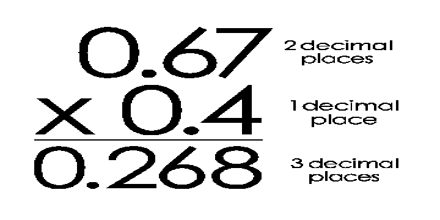Mathematic

# Multiplying Decimalsbasic objective of this lecture is to present on Multiplying Decimals. Here briefly describe on how to calculate Multiplying Decimals: Multiply normally, ignoring the decimal points. Then put the decimal point in the answer – it will have as many decimal places as the two original numbers combined. In other words, just count up how many numbers are after the decimal point in both numbers you are multiplying, then the answer should have that many numbers after its decimal point.